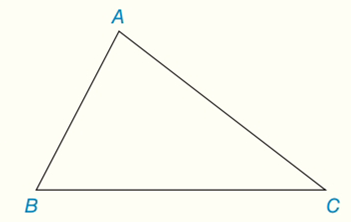Chapter 7.CT, Problem 7CTElementary Geometry For College St...

7th Edition
Alexander + 2 others
ISBN: 9781337614085

Solutions

Chapter
SectionElementary Geometry For College St...

7th Edition
Alexander + 2 others
ISBN: 9781337614085
Textbook Problem

For a given triangle (such as △ A B C ), what word describes the point of concurrency fora) the three perpendicular bisectors of sides?b) the three altitudes?To determine

(a)

To describe:

The point of concurrency for the three perpendicular bisectors of sides for a given triangle (such as ABC).

Explanation

Construct three perpendicular bisectors and they will cross each other at the point of concurrency called the circumcenter. A point where three or more lines intersect is called a point of concurrency. So, the circumcenter is the point of concurrency of perpendicular bisectors of a triangle. The point of concurrency is not necessarily inside the triangle. It may actually be in the triangle, on the triangle or outside of the triangle.

Calculation:

One of several centers the triangle can have, the circumcenter is the point where the perpendicular bisectors of a triangle intersect...

To determine

(b)

To describe:

The point of concurrency for the three altitudes for a given triangle (such as ABC).

Still sussing out bartleby?

Check out a sample textbook solution.

See a sample solution

The Solution to Your Study Problems

Bartleby provides explanations to thousands of textbook problems written by our experts, many with advanced degrees!

Get Started

Sketch the graphs of the equations in Exercises 512. xy=x2+1

Finite Mathematics and Applied Calculus (MindTap Course List)

Solve for x, assuming a, b, and c are negative constants. 60. ax+bcb

Single Variable Calculus: Early Transcendentals, Volume I

Multiply: 8374203_

Elementary Technical Mathematics

Add or subtract as indicated. 90(6225)

Trigonometry (MindTap Course List)

Evaluate i=1n(2i+2i).

Single Variable Calculus: Early Transcendentals

Sometimes, Always, or Never: If f(c) = 0, then c is a critical number.

Study Guide for Stewart's Single Variable Calculus: Early Transcendentals, 8th